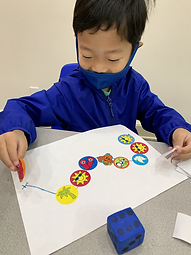## Ms. Kayla

### Target 1​

###### Lesson Type:

Continuation

Number Operation

:

Number Meaning

Understand the relationship between numbers and quantities.

###### 1:

Use one-to-one correspondence when counting to connect one quantity with one number name.

###### 2:

Count objects to determine how many there are.

Pre-K

###### Vocabulary:

Numbers, Dice, Count, Start, Finish

Activities:

1. Students used dice and stickers to practice one-to-one correspondence counting. Students rolled their dice and the number facing up was the one they counted. That number also determined how many stickers they could have. They then placed the stickers on their wavy line.### Home Exploration

###### Guiding Questions:## Absent Students:

### Target 2

:

###### 1:

Write numbers correctly.

###### 2:

Recognize a number without having to see its position in the count sequence.

Pre-K

###### Vocabulary:

Number, Trace, Puzzle

Activities:

1. Students practiced tracing numbers and saying the number names.

2. Students also completed small puzzles identifying the numeral, number name, and a picture.### Home Exploration

###### Guiding Questions:### Target 3

:

###### 1:

Identify simple geometric shapes in a variety of colors, sizes, and orientations.

###### 2:

Independently identify squares, rectangles, circles, and triangles.

Pre-K

###### Vocabulary:

Shapes, Tangram, Triangle, Square, Parallelogram, Turn

Activities:

1. Students used tangram shapes to construct an animal image.

2. Students identified individual geometric shapes when prompted by the teacher.### Home Exploration